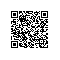# sql查找最小缺失值与重用被删除的键

create table tb_Test ( id int primary key, val char(1) null )

insert into tb_Test values(1,'a') insert into tb_Test values(2,'b') insert into tb_Test values(3,'c') insert into tb_Test values(4,'d') insert into tb_Test values(5,'e') insert into tb_Test values(6,'f') insert into tb_Test values(7,'g') insert into tb_Test values(8,'h')

delete from tb_Test where id in (1,2,4,5,7);select
case
when not exists(select 1 from tb_Test where id=1)

then 1
else (
select min(a.id+1)
from tb_Test as a
where not exists
(
select 1
from tb_Test as b
where b.id=a.id+1
)
)
end as '最小缺失值';

select Coalesce(Min(a.id+1),1)

from tb_Test a

where not exists (
select 1
from tb_Test as b
where b.id=a.id+1
) And exists(select 1 from tb_Test where id=1)使用钉钉扫一扫加入圈子
+ 订阅